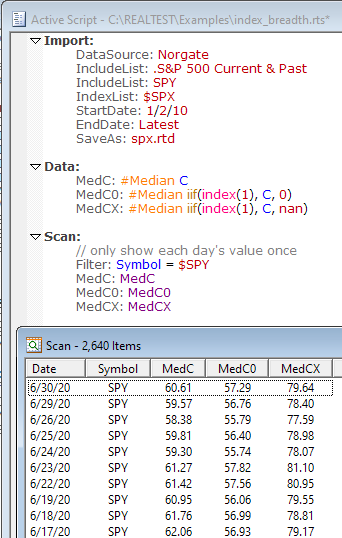Navigation: Realtest Script Language > Syntax Element Details > IsNan

Category

General-Purpose Functions

Description

Returns 1 (true) if formula can't be evaluated, or 0 (false) if it can.

Syntax

IsNan(value)

Parameters

value - formula

Notes

The most common reason that a formula can't be evaluated is that it tries to refer to more bars of data than are currently available. For example, if you import data for SPY starting 1/2/2010 and then try to calculate a 200-day moving average for 2/1/2010, there are not enough bars yet, so Avg(C,200) will return NAN, and IsNan(AVG(C,200)) will return TRUE.

Once any term of any formula evaluates to NAN, the result of the entire formula becomes NAN. This is why it can be useful to find out if a specific term is NAN before evaluating the entire formula. The IIF function is useful for this purpose.

The constant NAN can also be used in any formula (wherever a number is expected) to force the formula evaluation to fail. (NAN stands for "not a number".)

A great use for this NAN constant is when calculating a breadth function where you only want index constituents to be included for each date.

Say you want to know the median price per share of all stocks in the S&P 500 for each date in the past.

This example shows how to specify the Import, set up the Data section and run the Scan:Notice how each of the three columns shows different values.

MedC ignores index constituency so it includes all symbols that are no longer in the index (mostly penny stocks now).

MedC0 filters out non-constituents, but by substituting 0 for their prices, it skews the median value even lower than the the prior column.

MedX returns the correct values, because when a NAN is encountered by the cross-sectional calculator, that item is simply excluded from the calculation.